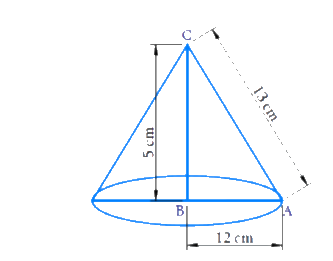# Ex.13.7 Q8 Surface Areas and Volumes Solution - NCERT Maths Class 9

## Question

If the triangle $$ABC$$ in the Question 7 above is revolved about the side $$5 \rm\,rm$$ then find the volume of the solid so obtained. Find also the ratio of the volumes of the two solids obtained in Questions 7 and 8.

Video Solution
Surface-Areas-And-Volumes
Ex exercise-13-7 | Question 8

## Text Solution

Reasoning:

Volume of the right circular cone is \begin{align}\frac{1}{3} \end{align}time of the volume of a cylinder\begin{align} = \frac{1}{3}\pi {r^2}h \end{align}.

What is  known?

Sides of the right triangle.

What is  unknown?

Volume of the cone and ratio between the volumes.

Solution:Radius of the cone $$= 12\rm\, cm$$

Height of the cone $$= 5 \rm\,cm$$

Volume of cone

\begin{align}&= \frac{1}{3}\pi {r^2}h \\ &= \frac{1}{3} \times \pi \times {(12)^2} \times 5 \\ & = 240\pi \,\,\,\rm\,c{m^3} \end{align}

Volume in question $$7$$\begin{align} = 100\pi \,\,\rm\,c{m^3} \end{align}

Ratio \begin{align}100:240 = 5:12 \end{align}

Answer:

Volume of the cone is \begin{align} = 240\pi \,\,\rm\,c{m^3} \end{align}

Ratio between the volume \begin{align}=5:12 \end{align}

Learn from the best math teachers and top your exams

• Live one on one classroom and doubt clearing
• Practice worksheets in and after class for conceptual clarity
• Personalized curriculum to keep up with school